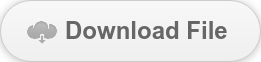Creep Analysis 1

One of the best examples to understand creep behavior was developed by Dr. El-Badry. This example is from Dr. El-Badry’s paper with some modifications.At time t0 = 7 days, the cantilever is subjected to a uniform load q = 0.225 klf (section is 12”×18”). At time t1 = 30 days, simple support is introduced at B, thus preventing the increase in deﬂection at B due to creep. Determine the end reaction RB at t2 = ∞. Ignore the diﬀerence between Ec(t0) and Ec(t1). RH (percent) is relative humidity and assumed 70%. f’c = 5 ksi (=34.47 MPa). L = 5ft.
First, calculate the creep coefficients based on CEB/FIP 90.h0 = 100mmIn the same way,Creep coefficient (t0 = 7 days)

Calculate modulus of elasticity.Modulus of ElasticityDeflections and rotations areThe restrained deflections/rotations for fixed end beam areApply this loading to the end supported cantilever, and we can get the following secondary reaction.The MIDAS output isThe differences are around 2%. The complete theory for creep calculation in MIDAS is not opened, and we do not know where these differences come from. However, considering the uncertainty of creep itself, the two outputs are close enough for practical design problems. Please download the MIDAS file below.Keep Updated With Technical Contents

Keeping You Updated

You might also like...#### Webinar: The Modeling and Comparison of a Curved Steel Plate Girder Bridge#### Expert Tip: Finite Element Method Basics 4#### Expert Tips: Dynamic Basics Part 2